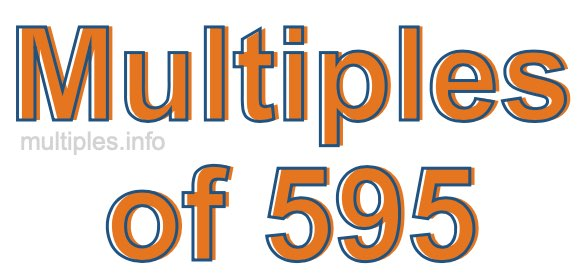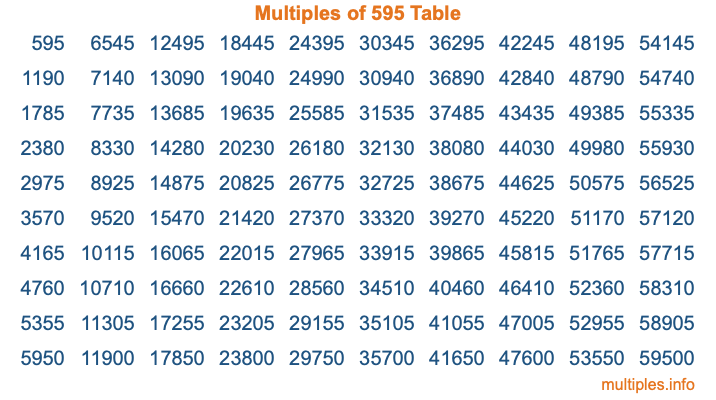Multiples of 595Welcome to the Multiples of 595 page. Here we will first teach you everything you will ever need to know about the multiples of 595, and then give you a study guide summary of everything we taught you to make sure you remember it all. Use this page to look up facts and learn information about the multiples of 595. This page will make you a multiples of five hundred ninety-five expert!

Definition of Multiples of 595
Multiples of 595 are all the numbers that when divided by 595 equal an integer. Each of the multiples of 595 are called a multiple. A multiple of 595 is created by multiplying 595 by an integer.

Therefore, to create a list of multiples of 595, you start with 1 multiplied by 595, then 2 multiplied by 595, then 3 multiplied by 595, and so on for as long as you want. Thus, the list of the first five multiples of 595 is 595, 1190, 1785, 2380, and 2975. To see a larger list of multiples of 595, see the printable image of Multiples of 595 further down on this page. We also have a category where you can choose any nth multiple of 595.

Multiples of 595 Checker
The Multiples of 595 Checker below checks to see if any number of your choice is a multiple of 595. In other words, it checks to see if there is any number (integer) that when multiplied by 595 will equal your number. To do that, we divide your number by 595. If the the quotient is an integer, then your number is a multiple of 595.

Is  a multiple of 595?

Least Common Multiple of 595 and ...
A Least Common Multiple (LCM) is the lowest multiple that two or more numbers have in common. This is also called the smallest common multiple or lowest common multiple and is useful to know when you are adding our subtracting fractions. Enter one or more numbers below (595 is already entered) to find the LCM.

Check out our LCM Calculator if you need more details about the Least Common Multiple or if you need the LCM for different numbers for adding and subtraction fractions.

nth Multiple of 595
As we stated above, 595 is the first multiple of 595, 1190 is the second multiple of 595, 1785 is the third multiple of 595, and so on. Enter a number below to find the nth multiple of 595.

th multiple of 595

Multiples of 595 vs Factors of 595
595 is a multiple of 595 and a factor of 595, but that is where the similarities end. All postive multiples of 595 are 595 or greater than 595. All positive factors of 595 are 595 or less than 595.

Below is the beginning list of multiples of 595 and the factors of 595 so you can compare:

Multiples of 595: 595, 1190, 1785, 2380, 2975, etc.

Factors of 595: 1, 5, 7, 17, 35, 85, 119, 595

As you can see, the multiples of 595 are all the numbers that you can divide by 595 to get a whole number. The factors of 595, on the other hand, are all the whole numbers that you can multiply by another whole number to get 595.

It's also interesting to note that if a number (x) is a factor of 595, then 595 will also be a multiple of that number (x).

Multiples of 595 vs Divisors of 595
The divisors of 595 are all the integers that 595 can be divided by evenly. Below is a list of the divisors of 595.

Divisors of 595: 1, 5, 7, 17, 35, 85, 119, 595

The interesting thing to note here is that if you take any multiple of 595 and divide it by a divisor of 595, you will see that the quotient is an integer.

Multiples of 595 Table
Below is an image of the first 100 multiples of 595 in a table. The table is in chronological order, column by column. The first column has the first ten multiples of 595, the second column has the next ten multiples of 595, and so on.The Multiples of 595 Table is also referred to as the 595 Times Table or Times Table of 595. You are welcome to print out our table for your studies.

Negative Multiples of 595
Although not often discussed or needed in math, it is worth mentioning that you can make a list of negative multiples of 595 by multiplying 595 by -1, then by -2, then by -3, and so on, to get the following list of negative multiples of 595:

-595, -1190, -1785, -2380, -2975, etc.

Multiples of 595 Summary
Below is a summary of important Multiples of 595 facts that we have discussed on this page. To retain the knowledge on this page, we recommend that you read through the summary and explain to yourself or a study partner why they hold true.

There are an infinite number of multiples of 595.

A multiple of 595 divided by 595 will equal a whole number.

595 divided by a factor of 595 equals a divisor of 595.

The nth multiple of 595 is n times 595.

The largest factor of 595 is equal to the first positive multiple of 595.

595 is a multiple of every factor of 595.

595 is a multiple of 595.

A multiple of 595 divided by a divisor of 595 equals an integer.

595 divided by a divisor of 595 equals a factor of 595.

Any integer times 595 will equal a multiple of 595.

Multiples of a Number
Here you can get the multiples of another number, all with the same attention to detail as we did for multiples of 595 on this page.

Multiples of
Multiples of 596
Did you find our page about multiples of five hundred ninety-five educational? Do you want more knowledge? Check out the multiples of the next number on our list!